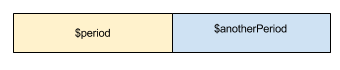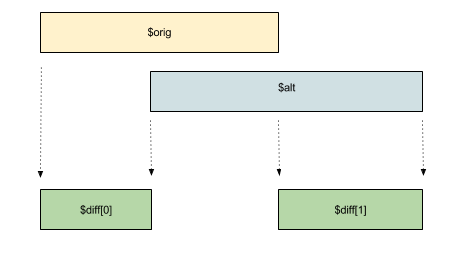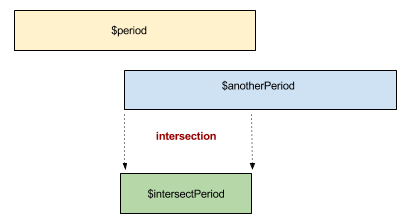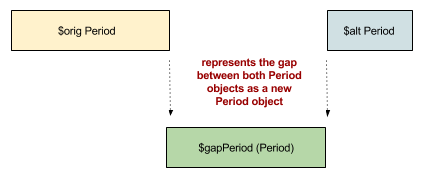# Period

## Versions

This is the documentation for `version 3.0` which will be supported until TBD. Please consider upgrading your code to the latest stable version

# Comparing Period objects

You can compare different `Period` objects according to their datepoints or durations.

## Using datepoints

### Period::isBefore

#### Description

``````<?php

public Period::isBefore(mixed \$index): bool
``````

Tells whether the current `Period` object datetime continuum is entirely before the specified `\$index`.

#### Parameter

The `\$index` argument can be another `Period` object or a datepoint.

#### Example

``````<?php

use League\Period\Period;

//comparing a datetime
\$period = Period::createFromMonth(1983, 4);
\$alt = Period::createFromMonth(1984, 4);
\$period->isBefore(\$alt); //returns true;
\$alt->isBefore(\$period); //return false;
``````

### Period::isAfter

#### Description

``````<?php

public Period::isBefore(mixed \$index): bool
``````

Tells whether the current `Period` object datetime continuum is entirely after the specified `\$index`.

#### Parameter

The `\$index` argument can be another `Period` object or a datepoint.

#### Example

``````<?php

use League\Period\Period;

//comparing a datetime
\$period = Period::createFromMonth(1983, 4);
\$alt = Period::createFromMonth(1984, 4);
\$alt->isAfter(\$period); //returns true;
\$period->isAfter(\$alt); //return false;
``````

### Period::abuts

#### Description

``````<?php

public Period::abuts(Period \$index): bool
``````

A `Period` abuts if it starts immediately after, or ends immediately before the submitted `Period` without overlapping.#### Example

``````<?php

use League\Period\Period;

\$period        = Period::createFromMonth(2014, 3);
\$anotherPeriod = Period::createFromMonth(2014, 4);
\$period->abuts(\$anotherPeriod); //return true
//in this case \$period->getEndDate() == \$anotherPeriod->getStartDate();
``````

### Period::overlaps

#### Description

``````<?php

public Period::overlaps(Period \$period): bool
``````

A `Period` overlaps another if they share some common part of their respective datetime continuum without abutting.

#### Example

``````<?php

use League\Period\Period;

\$orig  = Period::createFromMonth(2014, 3);
\$alt   = Period::createFromMonth(2014, 4);
\$other = Period::createFromDuration('2014-03-15', '3 WEEKS');

\$orig->overlaps(\$alt);   //return false
\$orig->overlaps(\$other); //return true
\$alt->overlaps(\$other);  //return true
``````

### Period::sameValueAs

#### Description

``````<?php

public Period::sameValueAs(Period \$period): bool
``````

Tells whether two `Period` objects shares the same datepoints.

#### Example

``````<?php

use League\Period\Period;

\$orig  = Period::createFromMonth(2014, 3);
\$alt   = Period::createFromMonth(2014, 4);
\$other = Period::createFromDuration('2014-03-01', '1 MONTH');

\$orig->sameValueAs(\$alt);   //return false
\$orig->sameValueAs(\$other); //return true
``````

### Period::contains

#### Description

``````<?php

public Period::contains(mixed \$index): bool
``````
• A `Period` contains a datepoint reference if this datepoint is present in its datetime continuum.
• A `Period` contains another `Period` object if the latter datetime continuum is completely contained within the `Period` datetime continuum.

#### Parameter

The `\$index` argument can be another `Period` object or a datepoint.

#### Example

``````<?php

use League\Period\Period;

//comparing a datetime
\$period = Period::createFromMonth(1983, 4);
\$period->contains('1983-04-15');      //returns true;
\$period->contains(\$period->getEndDate()); //returns false;

//comparing two Period objects
\$alt = Period::createFromDuration('1983-04-12', '12 DAYS');
\$period->contains(\$alt); //return true;
\$alt->contains(\$period); //return false;
``````

### Period::diff

#### Description

``````<?php

public Period::diff(Period \$period): array
``````

This method returns the difference between two `Period` objects only if they actually do overlap. If they do not overlap or abut, then an `Exception` is thrown.

The difference is expressed as an `array`. The returned array:

• is empty if both objects share the same datepoints;
• contains one `Period` object if both objects share only one datepoint;
• contains two `Period` objects if no datepoint are shared between objects. The first `Period` datetime continuum is always entirely set before the second one;#### Example

``````<?php

use League\Period\Period;

\$orig = Period::createFromDuration('2013-01-01', '1 MONTH');
\$alt  = Period::createFromDuration('2013-01-15', '7 DAYS');
\$diff = \$period->diff(\$alt);
// \$diff is an array containing 2 Period objects
// the first object is equal to new Period('2013-01-01', '2013-01-15');
// the second object is equal to new Period('2013-01-23', '2013-02-01');
\$diff->isBefore(\$diff); //return true;
//this is always true when two Period objects are present
``````

Before getting the difference, make sure the `Period` objects, at least, overlap each other.

### Period::intersect

#### Description

``````<?php

public function intersect(Period \$period): Period
``````

An Period overlaps another if it shares some common part of the datetime continuum. This method returns the amount of the overlap as a Period object, only if they actually do overlap. If they do not overlap or abut, then an Exception is thrown.

Before getting the intersection, make sure the `Period` objects, at least, overlap each other.#### Example

``````<?php

use League\Period\Period;

\$period        = Period::createFromDuration('2012-01-01', '2 MONTHS');
\$anotherPeriod = Period::createFromDuration('2012-01-15', '3 MONTHS');
\$intersectPeriod = \$period->intersect(\$anotherPeriod);
``````

### Period::gap

#### Description

``````<?php

public function gap(Period \$period): Period
``````

A `Period` has a gap with another Period if there is a non-zero interval between them. This method returns the amount of the gap as a new Period object only if they do actually have a gap between them. If they overlap a Exception is thrown.

Before getting the gap, make sure the `Period` objects do not overlaps.#### Example

``````<?php

use League\Period\Period;

\$orig = Period::createFromDuration('2012-01-01', '2 MONTHS');
\$alt  = Period::createFromDuration('2013-01-15', '3 MONTHS');
\$gapPeriod = \$orig->gap(\$alt);
``````

## Using durations

### Sorting objects

#### Description

``````<?php

public Period::compareDuration(Period \$period): int
public Period::durationGreaterThan(Period \$period): bool
public Period::durationLessThan(Period \$period): bool
public Period::sameDurationAs(Period \$period): bool
``````

The method `Period::compareDuration` compares two `Period` objects according to their duration. The method returns:

• `1` if the current object duration is greater than the submitted object duration;
• `-1` if the current object duration is lesser than the submitted object duration;
• `0` if the current object duration is equal to the submitted object duration;

To ease the method usage you can rely on the following proxy methods:

• `Period::durationGreaterThan` returns `true` when `Period::compareDuration` returns `1`
• `Period::durationLessThan` returns `true` when `Period::compareDuration` returns `-1`
• `Period::sameDurationAs` returns `true` when `Period::compareDuration` returns `0`

#### Examples

``````<?php

\$orig  = Period::createFromDuration('2012-01-01', '1 MONTH');
\$alt   = Period::createFromDuration('2012-01-01', '1 WEEK');
\$other = Period::createFromDuration('2013-01-01', '1 MONTH');

\$orig->compareDuration(\$alt);     //return 1
\$orig->durationGreaterThan(\$alt); //return true
\$orig->durationLessThan(\$alt);    //return false

\$alt->compareDuration(\$other);     //return -1
\$alt->durationLessThan(\$other);    //return true
\$alt->durationGreaterThan(\$other); //return false

\$orig->compareDuration(\$other);   //return 0
\$orig->sameDurationAs(\$other);    //return true
\$orig->sameValueAs(\$other);       //return false
//the duration between \$orig and \$other are equals but not the datepoints!!
``````

### Returning the duration differences

#### Description

``````<?php

public Period::dateIntervalDiff(Period \$period): DateInterval
public Period::timestampIntervalDiff(Period \$period): float
``````

Return the duration difference between two Period objects using a `DateInterval` object or expressed in seconds.

#### Examples

``````<?php

use League\Period\Period;

\$period    = Period::createFromSemester(2012, 1);
\$altPeriod = Period::createFromWeek(2012, 4);
\$diff = \$period->dateIntervalDiff(\$altPeriod);
// \$diff is a DateInterval object
\$diff_as_seconds = \$period->timestampIntervalDiff(\$altPeriod);
//\$diff_as_seconds represents the interval expressed in seconds
``````